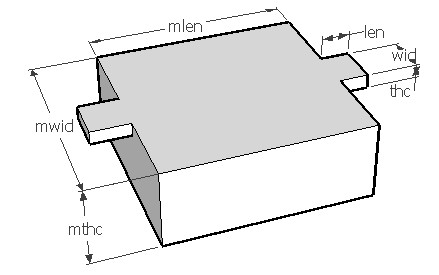CNTLMASS,len,wid,thc,mlen,mwid,mthc,den,ymod,sel
Vibration frequency of a clamped beam with a central mass

len          length of beam in µm
wid         width of beam in µm
thc          thickness of beam in µm
mlen       length of the mass in µm
mwid      width of the mass in µm
mthc       thickness of the mass in µm
den         density of the material in kg/m3
ymod      Young's modulus of the material in GPa
sel          number denoting the selected result.
Use 1 for normal vibration frequency, 2 for lateral vibration frequency and 3 for angular vibration frequencyNotes

An inertial mass suspended from two clamped beams is a structure often used in MEMS accelerometers and other motion sensitive devices. A typical structure is as shown above. It could be considered as a typical spring mass system. Three different vibration modes are discussed here. Vibration perpendicular to the plane of the mass, lateral vibration of the mass and a torsional or angular vibration of the mass about the beam axis are discussed. Use this form to estimate the vibration frequencies of normal, lateral and angular modes. The influence of the beam and mass dimensions on these modal frequencies could be understood.

The plot shows the amplitude frequency relationship for the given beam-mass. It shows the first resonance frequency as a sharp rise in amplitude. Using the cross hair tool, the resonant frequency and the corresponding relative amplitude can be obtained.

Assumptions

-The default material is Silicon with a Poisson's ratio of 0.27.
-The beam has uniform cross section.
-The weight of the beam and mass is uniformly distributed.
-There is no other load acting on the beam other than the weight of the mass and its own weight.
-The bending of the mass is negligible
-Damping is not involved.